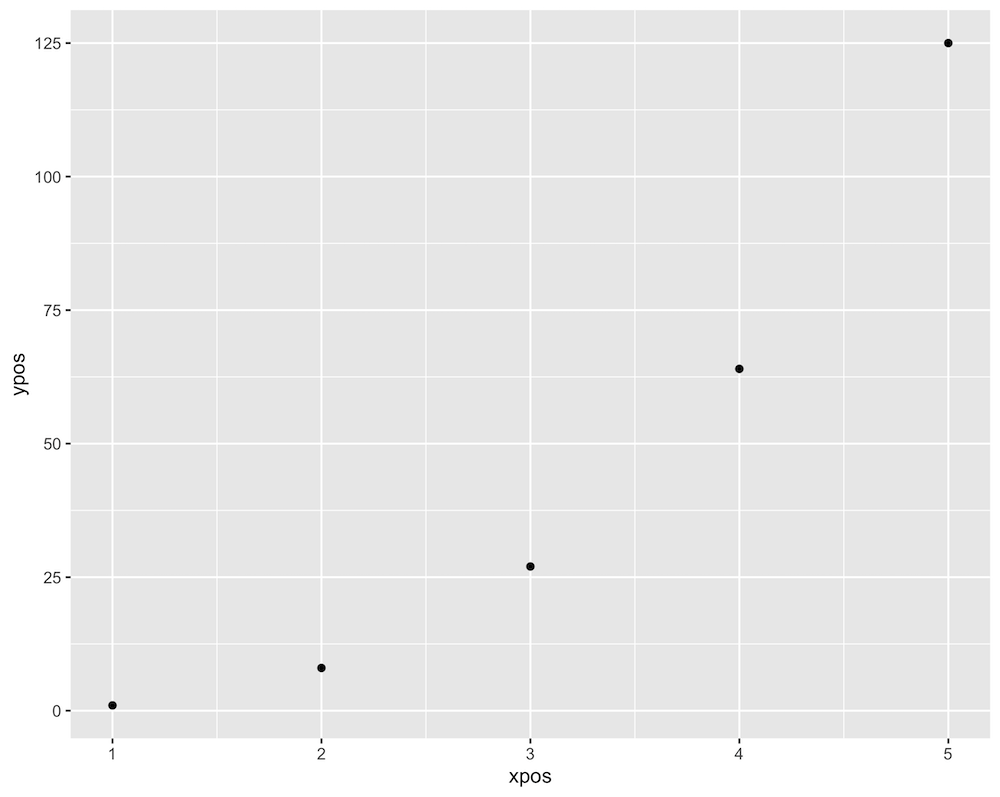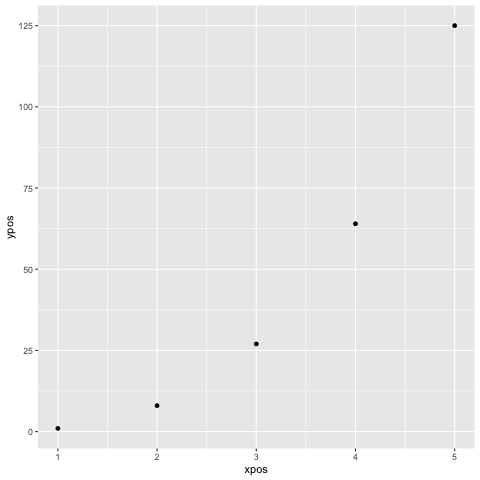# How to save a plot using ggplot2 in R?

• Last Updated : 17 Jun, 2021

In this article, we are going to see how to save GGPlot in R Programming language. ggplot2 is a plotting package in R that is used to create complex plots from data specified in a data frame. It provides a more programmatic interface for specifying which variables to plot on to the graphical device, how they are displayed, and general visual properties. The package can be installed and downloaded into the working space using the following syntax :

`install.packages("ggplot2")`

The ggplot() method is used to create different types of plots on the screen. It is used to bind a data frame to the data argument of the plot. It is used to also define the aesthetics and component mappings using the aes() attribute of the graph. It is used to create complex plots by selecting which variables are to be plotted and how to present them in the graph, for instance, the coordinates, shape, color, etc. The graphical representations can also be created in the form of geoms specific to the type of plot to be created.

`ggplot (data , mapping = aes(x, y)) + geom_point()`

### Method 1: Using ggsave()

The ggsave() is a method in the ggplot2 package which is used for saving the last plot that was displayed in the screen. It also makes assumption about the type of graphics device from the specified extension. The filename needs to be specified into the function call. The plot can be saved with both a .png or .pdf extension.

Syntax: ggsave( filename, plot = last_plot(), width = NA, height = NA, units = c(“in”, “cm”, “mm”),  path )

Arguments :

• filename – The character string name to assign to the plot
• plot – By default, it is used to save the last displayed plot.
• width, height, units – The dimensions of the plot
• path – The path to save the image can also be specified

Code:

## R

 `# importing the reqd libraries``library``(ggplot2)`` ` `# defining the x and y coordinates``xpos <- 1:5``ypos <- xpos**3`` ` `# creating a data frame``data_frame <- ``data.frame``(xpos = xpos, ypos = ypos)`` ` `# creating a plot``graph <- ``ggplot``(data_frame, ``aes``(xpos,ypos)) + ``  ``geom_point``()`` ` `# saving the plot as pdf ``ggsave``(``"cubegfg.pdf"``, graph, path = ``"/Users/mallikagupta/Desktop"``)`

Output

`Saving 7.47 x 5.96 in image`The plot can also be saved to the working space without using the ggsave() method. The png() method in R can be used to save a ggplot in the png format into the specified directory. The name of the plot along with the path name can be specified as an argument of the method.

`png(plot-path)`

### Method 2: Using dev.off()

The dev.off() method is then used to terminate this graphical device window and save the plot.

## R

 `# importing the reqd libraries``library``(ggplot2)`` ` `# defining the x and y coordinates``xpos <- 1:5``ypos <- xpos**3`` ` `# creating a data frame``data_frame <- ``data.frame``(xpos = xpos, ypos = ypos)`` ` `# creating a plot``graph <- ``ggplot``(data_frame, ``aes``(xpos,ypos)) + ``  ``geom_point``()``png``(``"Check.png"``)``print``(graph)``dev.off``()`

Output:My Personal Notes arrow_drop_up